## 6.1. Calculate Cp

Cp is an index used to assess the width of the process spread in comparison to the width of the specification. It is calculated by dividing the allowable spread by the actual spread. The allowable spread is the difference between the upper and lower specification limits. The actual spread is 6 times the estimated standard deviation. Plus or minus 3 times the estimated standard deviation contains 99.73 percent of the data and is commonly used to describe actual spread.

Cp for the example is: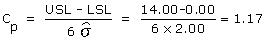A Cp of one indicates that the width of the process and the width of the specification are the same. A Cp of less than one indicates that the process spread is greater than the specification. This means that some of the data lies outside the specification. A Cp of greater than one indicates that the process spread is less than the width of the specification. Potentially this means that the process can fit inside the specification limits. The following diagrams show this graphically.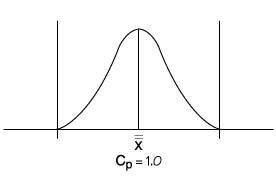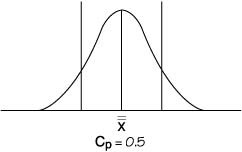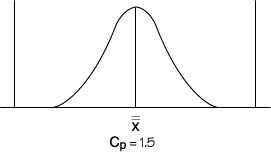In fact, the Cp states how many times the process can fit inside the specification. So a Cp of 1.5 means the process can fit inside the specification 1.5 times. A Cp greater than one is obviously desirable. However, the example has a Cp greater than one and yet it still has data outside the specification. This is due to the position of the overall average relative to the specification. When the overall average is away from the center of the specification, the system can still produce data outside the specification even though the Cp is greater than one, as in the example below: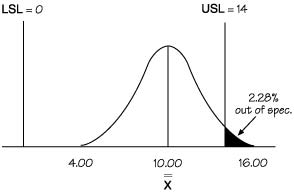To overcome this problem, Cpk was created.

The above article is an excerpt from the “Operational definition” chapter of Practical Tools for Continuous Improvement Volume 2 Statistical Tools.